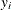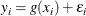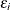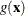### Local Regression and the Loess Method

Assume that for i = 1 to n, the ith measurementof the response y and the corresponding measurementof the vectorof p predictors are related bywhere g is the regression function andis a random error. The idea of local regression is that at a predictor, the regression functioncan be locally approximated by the value of a function in some specified parametric class. Such a local approximation is obtained by fitting a regression surface to the data points within a chosen neighborhood of the point.

In the loess method, weighted least squares is used to fit linear or quadratic functions of the predictors at the centers of neighborhoods. The radius of each neighborhood is chosen so that the neighborhood contains a specified percentage of the data points. The fraction of the data, called the smoothing parameter, in each local neighborhood controls the smoothness of the estimated surface. Data points in a given local neighborhood are weighted by a smooth decreasing function of their distance from the center of the neighborhood.

In a direct implementation, such fitting is done at each point at which the regression surface is to be estimated. A much faster computational procedure is to perform such local fitting at a selected sample of points in predictor space and then to blend these local polynomials to obtain a regression surface.

You can use the LOESS procedure to perform statistical inference provided that the error distribution satisfies some basic assumptions. In particular, such analysis is appropriate when theare iid normal random variables with mean 0. By using the iterative reweighting, the LOESS procedure can also provide statistical inference when the error distribution is symmetric but not necessarily normal. Furthermore, by doing iterative reweighting, you can use the LOESS procedure to perform robust fitting in the presence of outliers in the data.

While all output of the LOESS procedure can be optionally displayed, most often the LOESS procedure is used to produce output data sets that will be viewed and manipulated by other SAS procedures. PROC LOESS uses the Output Delivery System (ODS) to place results in output data sets. Alternatively, PROC LOESS also provides an OUTPUT statement to create SAS data sets from analysis results.